### GATE 2014 ECE Video Solutions on Analog Circuits (Analog Electronics)

Set – 1 (15th February 2014 (Forenoon))

1. In the figure, assume that the forward voltage drops to the PN diode D1 and Schottky diode D2 are 0.7 volts and 0.3 volts respectively. If ON denotes conducting state of the diode and OFF denotes the non conducting state of the diode, then in the circuit,a. Both are ON
b. D1 is ON and D2 is OFF
c. Both are OFF
d. D1 is OFF and D2 is ON

2. A good current buffer has
a. Low input impedance and low output impedance
b. Low input impedance and high output impedance
c. high input impedance and low output impedance
d. high input impedance and high output impedance

3. In the ac equivalent circuit shown in the figure, if iin is the input current and RF is very large, then the type of feedback isa. Voltage – Voltage feedback
b. Voltage – Current feedback
c. Current – Voltage feedback
d. Current – Current feedback

4. In the low pass filter shown in the figure, for a cutoff frequency of 5 KHz, the value of R2 (in KΩ) is ……..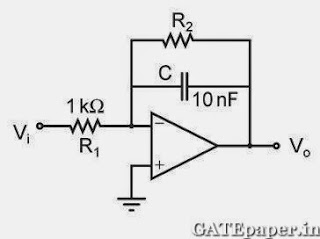5. A BJT is biased in forward active mode. Assume VBE = 0.7 volts, KT/q = 25 mV and reverse saturation current IS = 10-13 Amp. The Transconductance of the BJT (in mA/volt) is…..

6. In the voltage regulator circuit shown in the figure, the Op-Amp is ideal. The BJT has VBE = 0.7 volts, and β = 100, and the zener voltage is 4.7 volts. For a regulated output of 9 volts, the value of R (in Ω) is ……..7. In the circuit shown, the Op-Amp has finite input resistance, infinite voltage gain and zero input offset voltage. The output voltage Vout is …….a. – I2(R1 + R2)
b. I2R2
c. I1R2
d. – I1(R1 + R2)

8. For the amplifier shown in the figure, the BJT parameters are VBE = 0.7 volts, β = 200, and thermal voltage VT = 25 mV. The voltage gain (vo/vi) of the amplifier is ……..SET – 2 (15th February 2014 (Afternoon))

1. The diode in the circuit shown, if Von = 0.7 volts but is ideal otherwise. If Vi = 5 sin(ωt) volts, the minimum and maximum values of Vo (in volts) are, respectively,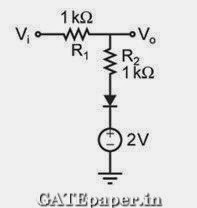a. -5 and 2.7
b. 2.7 and 5
c. -5 and 3.85
d. 1.3 and 5

2. The feedback topology in the amplifier circuit (the base bias circuit is not shown for simplicity) in the figure isa. Voltage – Shunt feedback
b. Current – Series feedback
c. Current – Shunt feedback
d. Voltage – Series feedback

3. In the differential amplifier shown in the figure, the magnitude of the common mode and differential mode gains are Acm and Ad, respectively. If the resistance RE is increased, thena. Acm increases
b. Common mode rejection ratio increases
d. Common mode rejection ratio decreases

4. A cascade connection of two voltage amplifiers A1 and A2 is shown in the figure. The open loop gain Avo, input resistance Rin, and output resistance Ro for A1 and A2 are as follows:
A1 : Avo = 10, Rin = 10 KΩ, Ro = 1 KΩ
A2 : Avo = 5, Rin = 5 KΩ, Ro = 200 Ω
The approximate overall voltage gain Vout / Vin is …………….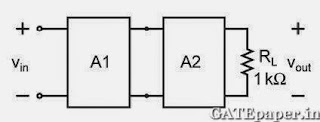SET – 3 (16th February 2014 (Forenoon))

1. In the circuit shown, the PNP transistor has |VBE| = 0.7 volts and β = 50. Assume that RB = 100 KΩ. For Vo to be 5 volts, the value of RC (in KΩ) is ……..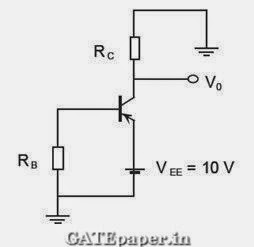2. The desirable characteristics of a Transconductance amplifier are
a. High input resistance and High output resistance
b. High input resistance and Low output resistance
c. Low input resistance and High output resistance
d. Low input resistance and Low output resistance

3. The figure shows a half wave rectifier. The diode D is ideal. The average steady state current (in Amperes) through the diode is approximately…………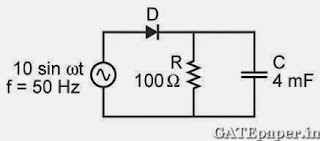4. Assuming that the Op-Amp in the circuit is ideal, then the output voltage is5. In the circuit shown, the silicon BJT has β = 50. Assume VBE = 0.7 volts and VCEsat = 0.2 volts. Which one of the following statements is correct?a. For RC = 1 kΩ, the BJT operates in the saturation region
b. For RC = 3 kΩ, the BJT operates in the saturation region
c. For RC = 20 kΩ, the BJT operates in the cutoff region
d. For RC = 20 kΩ, the BJT operates in the linear region

SET - 4 (16th February 2014 (Afternoon))
1. If the emitter resistance in a common emitter voltage amplifier is not bypassed, it will
a. Reduce both the voltage gain and the input impedance
b. Reduce the voltage gain and increase the input impedance
c. Increase the voltage gain and reduce the input impedance
d. Increase both the voltage gain and the input impedance

2. Consider two BJTs biased at the same collector current with area A1 = 0.2 µm x 0.2 µm and A2 = 300 µm x 300 µm. assuming that all other device parameters are identical, KT/q = 26 mV, the intrinsic carrier concentration is 1 x 1010 cm-3, and q = 1.6 x 10-19C, the difference between the base emitter voltages (in mV) of the two BJTs (i.e. VBE1 – VBE2) is …………….

3. A BJT in a common base configuration is used to amplify a signal received by a 50Ω antenna. Assume KT/q = 25 mV, the value of collector bias current (in mA) required to match the input impedance of the amplifier to the impedance of the antenna is ………..

4. Two silicon diodes, with a forward voltage drop of 0.7 volts, are used in the circuit shown in the figure. The range of input voltage Vi for which the output voltage Vo = Vi, is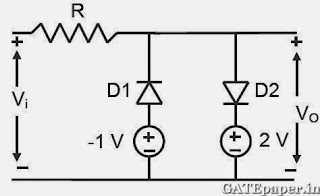a. -0.3 volts < Vi < 1.3 volts
b. -0.3 volts < Vi < 2 volts
c. -1.0 volts < Vi < 2.0 volts
d. -1.7 volts < Vi < 2.7 volts

5. The circuit represents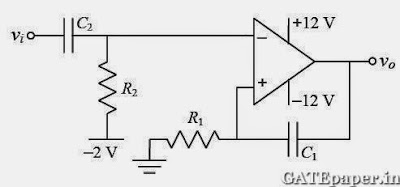a. A band pass filter
b. A voltage controlled oscillator
c. An Amplitude modulator
d. A monostable multivibrator

6. Consider the common collector amplifier in the figure (bias circuitry ensures that the transistor operates in forward active region, but has been omitted for simplicity). Let IC be the collector current, VBE be the base emitter voltage and VT be the thermal voltage. Also, gm and ro are the small signal Transconductance and output resistance of the transistor, respectively. Which one of the following conditions ensures a nearly constant small signal voltage gain for a wide range of values of RE?7. For the common collector amplifier shown in the figure, the BJT has high β, negligible VCEsat and VBE = 0.7 volts. The maximum undistorted peak to peak output voltage Vo (in volts) is ………1.thank u very much

2.This is my first time i visit here. I found so many interesting stuff in your blog especially its discussion. From the tons of comments on your articles, I guess I am not the only one having all the enjoyment here! keep up the good work ECE R100

3.n the circuit in Figure 6.77 the transistor parameters are: β = 80, VB E (on) = 0.7 V, and VA = ∞.
Determine the average power dissipated in RC, RL, and Q for: (a) vs = 0, and (b) vs = 18 cos ωt mV.

4.http://www.slowfoodogliopo.it/tttt/vixo-f-inter-juve-X-t-b-03.html
http://www.slowfoodogliopo.it/tttt/vixo-f-inter-juve-X-t-b-01.html
http://www.slowfoodogliopo.it/tttt/vixo-f-inter-juve-X-s-b-02.html
http://www.slowfoodogliopo.it/tttt/vixo-f-inter-juve-X-p-b-04.html
http://www.slowfoodogliopo.it/tttt/vixo-f-inter-juve-X-n-b-05.html

5.https://rusmi.su/fgf/get/video-c-v-bz1.html
https://rusmi.su/fgf/get/video-c-v-bz2.html
https://rusmi.su/fgf/get/video-c-v-bz3.html
https://rusmi.su/fgf/get/video-c-v-bz4.html
https://rusmi.su/fgf/get/video-c-v-bz5.html

6.https://rusmi.su/fgf/get/video-g-v-ni1.html
https://rusmi.su/fgf/get/video-g-v-ni2.html
https://rusmi.su/fgf/get/video-g-v-ni3.html
https://rusmi.su/fgf/get/video-g-v-ni4.html
https://rusmi.su/fgf/get/video-g-v-ni5.html
https://rusmi.su/fgf/get/video-g-v-ni6.html
https://rusmi.su/fgf/get/video-a-v-fi1.html
https://rusmi.su/fgf/get/video-a-v-fi2.html
https://rusmi.su/fgf/get/video-a-v-fi3.html
https://rusmi.su/fgf/get/video-a-v-fi4.html

7.https://rusmi.su/fgf/get/video-b-v-a1.html
https://rusmi.su/fgf/get/video-b-v-a2.html
https://rusmi.su/fgf/get/video-b-v-a3.html
https://rusmi.su/fgf/get/video-b-v-a4.html
https://rusmi.su/fgf/get/video-b-v-a5.html
https://rusmi.su/fgf/get/video-b-v-a6.html
https://rusmi.su/fgf/get/video-b-v-a7.html
https://rusmi.su/fgf/get/video-b-v-a8.html
https://rusmi.su/fgf/get/video-b-v-a9.html

8.Q: Construct the Circuit showing in Figure below using Multism14. Then find the
DC and AC output voltage with filter (Key closed) and without filter. Note: The Key
(K) connected in series with Cr .shows your Results as in tablel. Where NI/N2:
10/1 , Vin :230Vrms.Compare your results with theoretical value for DC
output voltage without filter. Draw the ripple voltage (AC) ofthe output for R:lOK
and C;10pF using Oscilloscope(take image).

Name

Email *

Message *